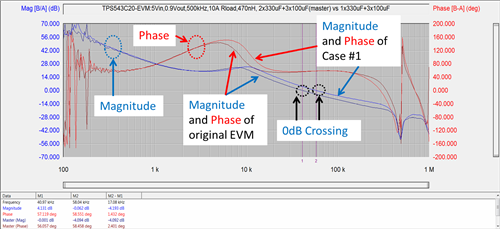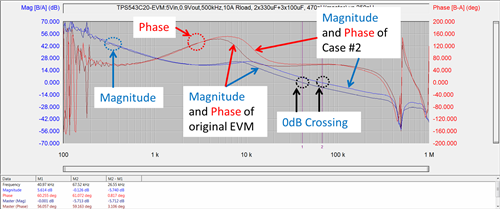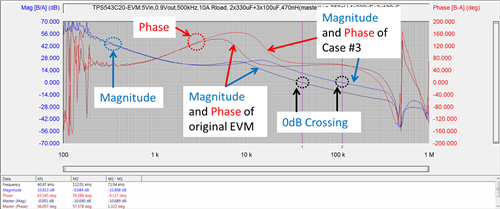# Maintain a constant phase margin in a synchronous buck converter

Other Parts Discussed in Post: TPS543C20

When designing a step-down buck converter, converter stability should be a top priority. The converter is stable when the phase margin of the loop gain is greater than 0 degrees, with an acceptable minimum phase margin of 45 degrees.  Maintaining a constant phase margin when the power-stage components vary as much as 50% from their original values can be a challenge for circuit designers.  For example, the values of the inductor and capacitors change with their bias operating conditions and temperature range.

Using the new advanced current mode (ACM) control topology in TI’s TPS543C20 synchronous step-down SWIFT™ converter, I compared the shape of the phase in three separate cases. In each case, I change the value of the output capacitor, inductor and both the output capacitor and inductor without making any other modifications to the circuit. I used the single-phase TPS543C20EVM-799 evaluation module (EVM) to perform the comparison under these test conditions:

• Input voltage = 5V, output voltage = 0.9V, switching frequency = 500kHz.
• Original inductor value = 470nH (0.165mΩ direct current resistance [DCR]).
• Original output capacitor = 2 x 330µF (3mΩ equivalent series resistance [ESR]) + 3 x 100µF (1206 size ceramic capacitor).
• Output load configured as a 10A resistive power resistor.

Case No. 1, illustrated in Figure 1

I compared the phase from the original default EVM to a new output capacitance value of 1 x 330µF (3mΩ ESR) + 3 x 100µF while keeping other conditions the same. As you can see, the shape of the phase very much stays the same as the original values. The phase is also basically staying constant over a decade of frequency after the double-pole frequency of inductor and output capacitor.Figure 1: Bode plot comparison between original configurations versus case No. 1

Case No. 2, illustrated in Figure 2

I compared the phase of the original default EVM to a new inductor value – 250nH (0.165mΩ DCR) – while keeping the other conditions the same. Again, the shape of the phase is very much the same as the default configuration. The phase is basically staying constant over a decade of frequency.Figure 2: Bode plot comparison between original configurations versus case No. 2

Case No. 3, illustrated in Figure 3

I compared the phase of the original default EVM to a combination of case Nos. 1 and 2 – 250nH (0.165mΩ DCR) and 1 x 330µF (3mΩ ESR) + 3 x 100µF – while keeping the other conditions the same. Again, the shape of the phase stays constant at 0dB.Figure 3: Bode plot comparison between original configurations versus case No. 3

The shape of the phase in the ACM topology stays constant over a decade frequency range when the power component changes its value, such as a 53% inductor reduction from 470nH to 250nH.  However, you still need to pay attention to the changing value of power-stage components so that the phase margin of your converter meets the minimum requirement.